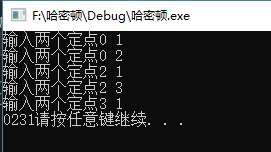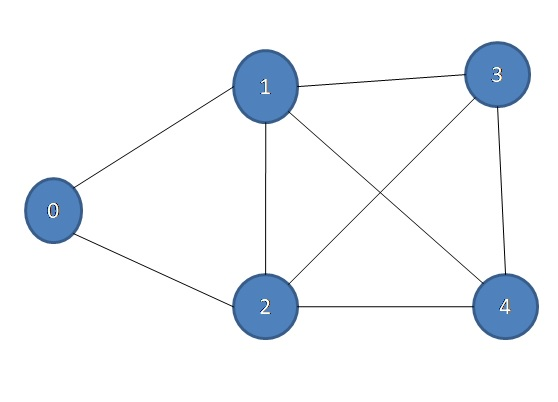• （2）设计算法判断图G是否存在哈密顿路径，如果存在，输出一天哈密顿路径即可； （3）分析算法的时间复杂度。 3、设计思想 寻找哈密顿路径的过程是一个深度优先遍历的过程。在遍历过程中，如果有回溯，说明遍历...

不要自卑，去提升实力 互联网行业谁技术牛谁是爹 如果文章可以带给你能量，那是最好的事！请相信自己 加油o~

1、问题描述

在图G中找出一条包含所有顶点的简单路径，该路径称为哈密顿路径

2、基本要求

（1）图G是非完全有向图，且图G不一定存在哈密顿路径； （2）设计算法判断图G是否存在哈密顿路径，如果存在，输出一天哈密顿路径即可； （3）分析算法的时间复杂度。

3、设计思想

寻找哈密顿路径的过程是一个深度优先遍历的过程。在遍历过程中，如果有回溯，说明遍历经过的路线中存在重复访问的节点，所以，可以修改深度优先遍历算法，使其在遍历过程中取消回溯。

以下代码仅供参考 以下代码仅供参考 以下代码仅供参考
/**
*作者：魏宝航
*2020年11月22日,下午21:47
*/
import java.io.IOException;
import java.util.Scanner;

public class MatrixUDG {

private char[] mVexs;
private int[][] mMatrix;
private int num;
private int edge;
private boolean[] visit;
private static int count=0;
private int m=0;

public MatrixUDG(char[] vexs, char[][] edges) {

num = vexs.length;
edge = edges.length;

visit=new boolean[num+10];

mVexs = new char[num];
for (int i = 0; i < mVexs.length; i++)
mVexs[i] = vexs[i];

mMatrix = new int[num][num];
for(int i=0;i<edge;i++){
for(int j=0;j<edge;j++){
if(edges[i][j]=='∞'){
mMatrix[i][j]=Integer.MAX_VALUE;
}
else{
mMatrix[i][j]=1;
}
}
}
}

public void print() {
System.out.printf("Martix Graph:\n");
for (int i = 0; i < mVexs.length; i++) {
for (int j = 0; j < mVexs.length; j++){
if(mMatrix[i][j]<Integer.MAX_VALUE){
System.out.printf("%d ", mMatrix[i][j]);
}else{
System.out.print("∞ ");
}
}

System.out.printf("\n");
}
}

public void DFS(int v){
visit[v]=true;
for(m=0;m<num;m++){
if(mMatrix[v][m]==1&&visit[m]==false){
DFS(m);
}
}
if(m==num){
visit[v]=false;
count--;
}
}

public void Hamilton(){
for(int i=0;i<num;i++){
DFS(i);
}
for(int i=1;i<num;i++){
}
}

public static void main(String[] args) {

char[] vexs2={'1','2','3','4'};
char[][] edges2=new char[][]{
{'∞','1','1','∞'},
{'∞','∞','∞','∞'},
{'∞','1','∞','1'},
{'∞','1','∞','∞'},
};

MatrixUDG g=new MatrixUDG(vexs2,edges2);
g.print();
g.Hamilton();
}
}

展开全文数据结构 java dfs leetcode
• ## 哈密顿路径

千次阅读 2019-01-10 12:33:50
这个“哈密瓜路径”网上查了好久没搞明白，我这个的代码无奈定义了4个节点和5条边，将就混过课程设计课。...（2）设计算法判断图G是否存在哈密顿路径，如果存在，输出一条哈密顿路径即可； （3）分析算法...
这个“哈密瓜路径”网上查了好久没搞明白，我这个的代码无奈定义了4个节点和5条边，将就混过课程设计课。
放代码和结果从我做起！欢迎大家留言！
1.  问题描述
在图G中找出一条包含所有顶点的简单路径，该路径称为哈密顿路径。
2. 基本要求
（1）图G是非完全有向图，且图G不一定存在哈密顿路径；
（2）设计算法判断图G是否存在哈密顿路径，如果存在，输出一条哈密顿路径即可；
（3）分析算法的时间复杂度。
3. 问题分析
哈密顿路径也称作哈密顿链，指在一个图中沿边访问每个顶点恰好一次的路径。寻找这样的一个路径是一个典型的NP-完全(NP-complete)问题。图中有的边可以不经过,但是不会有边被经过两次。与欧拉图的区别:欧拉图讨论的实际上是图上关于边的可行便利问题,而哈密顿图的要求与点有关。
判定：
一．Dirac定理(充分条件)
设一个无向图中有N个顶点,若所有顶点的度数大于等于N/2,则哈密顿回路一定存在(N/2指的是向上取整)。
二．基本的必要条件
设图G=<V, E>是哈密顿图,则对于v的任意一个非空子集S,若以|S|表示S中元素的数目,G-S表示G中删除了S中的点以及这些点所关联的边后得到的子图,则W(G-S)<=|S|成立。其中W(G-S)是G-S中联通分支数。
三．竞赛图(哈密顿通路)
N(N>=2)阶竞赛图一点存在哈密顿通路。
4. 概要设计
在Dirac定理的前提下构造哈密顿回路的过程:
1）任意找两个相邻的节点S和T,在其基础上扩展出一条尽量长的没有重复结点的路径。即如果S与结点v相邻,而且v不在路径S -> T上,则可以把该路径变成v -> S -> T,然后v成为新的S。从S和T分别向两头扩展,直到无法继续扩展为止,即所有与S或T相邻的节点都在路径S -> T上。
2）若S与T相邻,则路径S -> T形成了一个回路。
3） 若S与T不相邻,可以构造出来一个回路.设路径S -> T上有k+2个节点,依次为S, v1, v2, ..., vk, T.可以证明存在节点vi(i属于[1, k]),满足vi与T相邻,且vi+1与S相邻.找到这个节点vi,把原路径变成S -> vi -> T -> vi+1 ,即形成了一个回路。
4）到此为止,已经构造出来了一个没有重复节点的的回路,如果其长度为N,则哈密顿回路就找到了。如果回路的长度小于N,由于整个图是连通的,所以在该回路上,一定存在一点与回路之外的点相邻。那么从该点处把回路断开,就变回了一条路径,同时还可以将与之相邻的点加入路径。再按照步骤1的方法尽量扩展路径,则一定有新的节点被加进来。接着回到路径2。
证明:
根据鸽巢定理，既然与S和T相邻的点都在路径上，它们分布的范围只有v1,v2，---,vk这k个点，k<=N-2，跟据哈密顿回路的第一个判断条件，d(S)+d(T)>=N，那么v1,v2,---，vk这k个点中一定有至少2个点同时与S和T相连，根据鸽巢定理，肯定存在一个与S相邻的点vi和一个与T相邻的点vj，满足j=i+1。
哈密顿路径的伪代码如下:
算法：哈密顿路径
输入：哈密顿回路的起始点s,哈密顿回路中终点s之前的点t
输出：最终的哈密顿回路ans[ ]
1.初始化,令s = 1,t为s的任意一个邻接点;
2.如果ans[]中元素的个数小于n,则从t开始向外扩展,如果有可扩展点v,放入ans[]的尾部,并且t=v,并继续扩展,如无法扩展进入步骤3;
3.将当前得到的ans[]倒置,s和t互换,从t开始向外扩展,如果有可扩展点v,放入ans[]尾部,并且t=v,并继续扩展。如无法扩展进入步骤4;
3.1如果当前s和t相邻,进入步骤5。否则,遍历ans[],寻找点ans[i],使得ans[i]与t相连并且ans[i +１]与ｓ相连,将从ans[i + 1]到t部分的ans[]倒置,t=ans[i +１],进如步骤5;
3.2如果当前ans[]中元素的个数等于n,算法结束,ans[]中保存了哈密顿回路(可看情况是否加入点s).否则,如果s与t连通,但是ans[]中的元素的个数小于n,则遍历ans[],寻找点ans[i],使得ans[i]与ans[]外的一点(j)相连,则令s=ans[i - 1],t = j,将ans[]中s到ans[i - 1]部分的ans[]倒置,将ans[]中的ans[i]到t的部分倒置,将点j加入到ans[]的尾部,转步骤2;

时间复杂度:
如果说每次到步骤5算一轮的话,那么由于每一轮当中至少有一个节点被加入到路径S -> T中,所以总的轮数肯定不超过n轮,所以时间复杂度为O(n^2).空间上由于边数非常多,所以采用邻接矩阵来存储比较适合。
5. 编程结果
#include<stdio.h>
#include<stdlib.h>
#define Maxsize 10
#define MAX_NO_LIMIT 10000000
typedef char Datatype;
int visited[Maxsize] = { 0 };
int s[Maxsize] = { 0 };
int count = 0;
typedef struct
{
Datatype vertex[Maxsize];
int edge[Maxsize][Maxsize];
int vertexnum, edgenum;

}graph;
void creatgraph(graph *G, Datatype a[], int n, int e)
{
int i, j, k;
G->vertexnum = n;
G->edgenum = e;
for (i = 0; i<G->vertexnum; i++)
{
G->vertex[i] = a[i];
}
for (i = 0; i<G->vertexnum; i++)
{
for (j = 0; j<G->vertexnum; j++)
{
G->edge[i][j] = 0;
}
}
for (k = 0; k<G->edgenum; k++)
{
printf("输入两个定点");
scanf_s("%d%d", &i, &j);
G->edge[i][j] = 1;
G->edge[j][i] = MAX_NO_LIMIT;
}

}
void DFS(graph *G, int v)          //count是全局变量并已初始化为0
{
int j;
visited[v] = 1; //对访问点进行标记
s[count++] = v;//S表示路径，这里为开头为v
for (j = 0; j <G->vertexnum; j++) {
if (G->edge[v][j] == 1 && visited[j] == 0)
DFS(G, j); //这里对点进行递归
}
if (j == G->vertexnum) {   //取消回溯
visited[v] = 0;
count--;
}
}
int main()
{
int i;
char ch[] = { 'a','b','c','d' };
graph MG;
creatgraph(&MG, ch, 4, 5);
DFS(&MG, 0);
for (i = 0; i<4; i++)
{
printf("%d",s[i]);
}
system("pause");
return 0;
}展开全文• 哈密顿路径Problem Statement: 问题陈述： Given a graph G. you have to find out that that graph is Hamiltonian or not. 给定图G。 您必须找出该图是否为哈密顿量 。 Example: 例： Input: 输入： ...

哈密顿路径
Problem Statement:
问题陈述：
Given a graph G. you have to find out that that graph is Hamiltonian or not.
给定图G。 您必须找出该图是否为哈密顿量 。
Example:
例：
Input:
输入：Output: 1
输出1
Because here is a path 0 → 1 → 5 → 3 → 2 → 0 and 0 → 2 → 3 → 5 → 1 → 0
因为这里是路径0→1→5→3→2→0和0→2→3→5→1→0
Algorithm:
算法：
To solve this problem we follow this approach:
为了解决这个问题，我们采用以下方法：
We take the source vertex and go for its adjacent not visited vertices. 我们获取源顶点，并寻找其相邻的未访问顶点。 Whenever we find a new vertex we make it source vertex and we repeat step 1. 每当我们找到一个新顶点时，就将其设为源顶点，然后重复步骤1。 When a vertex count is equal to the vertex number then we check that from vertex is there any path to the source vertex. If there is exist a path to the source vertex then we will mark it and if not then make that vertex as unmarked and continue the process. 当顶点数等于顶点数时，我们检查从顶点开始是否存在到源顶点的任何路径。 如果存在到源顶点的路径，那么我们将对其进行标记，如果没有，则将该顶点设为未标记并继续该过程。 If there is no such path then we say NO. 如果没有这样的路径，那么我们说不。

.minHeight{
min-height: 250px;
}
@media (min-width: 1025px){
.minHeight{
min-height: 90px;
}
}

.minHeight{
min-height: 250px;
}
@media (min-width: 1025px){
.minHeight{
min-height: 90px;
}
}

检查图是否为哈密顿的C ++实现 (C++ Implementation to check a graph is Hamiltonian or not )
#include <iostream>
#include <bits/stdc++.h>
using namespace std;

void addedge(list<int>* g, int u, int v)
{
g[u].push_back(v);
g[v].push_back(u);
}

int hamiltonian(list<int>* g, int v, int s, int& count, bool* vis, int& h)
{
if (count > 1 && s == 0) {
h = 1;
return 1;
}
list<int>::iterator it;
for (it = g[s].begin(); it != g[s].end(); it++) {
if (!vis[*it]) {
vis[*it] = true;
count++;
if (count == v) {
vis = false;
}
hamiltonian(g, v, *it, count, vis, h);
vis = true;
vis[*it] = false;
count--;
}
}
return 0;
}

int main()
{
int num;
cin >> num;
for (int i = 0; i < num; i++) {
int v, e;
cin >> v >> e;
list<int> g[v];
int x, y;
for (int j = 0; j < e; j++) {
cin >> x >> y;
}
bool vis[v];
memset(vis, false, sizeof(vis));
int count = 1;
vis = true;
int h = 0;
hamiltonian(g, v, 0, count, vis, h);
cout << h << endl;
}
return 0;
}


Output
输出量
1
5
8
0 1
0 2
1 2
1 3
1 4
3 4
3 2
2 4
1


翻译自: https://www.includehelp.com/icp/check-a-graph-is-hamiltonian-or-not-hamiltonian-path.aspx

哈密顿路径

展开全文java python leetcode php
• 转自：汉密尔顿路径（哈密顿路径）解析 汉密尔顿路径（哈密顿路径哈密顿路径也称作哈密顿链，指在一个图中沿边访问每个顶点恰好一次的路径。寻找这样的一个路径是一个典型的NP-完全(NP-complete）问题。后来人们...


转自：汉密尔顿路径（哈密顿路径）解析
汉密尔顿路径（哈密顿路径）
哈密顿路径也称作哈密顿链，指在一个图中沿边访问每个顶点恰好一次的路径。寻找这样的一个路径是一个典型的NP-完全(NP-complete）问题。后来人们也证明了，找一条哈密顿路的近似比为常数的近似算法也是NP完全的.
算法思路（寻找图中所有的哈密顿路）
首先用一个邻接矩阵存储图将每一个顶点作为起点，查找哈密顿路查找哈密顿路的思路：采用递归遍历的方式在递归的搜索的过程中同时需要不断的修改边可以链接的状态。0不可以链接 1 可以链接。
findHamiltonpath(int[][] M, int x, int y, int l) {
int i;
for (i = x; i < len; i++) { // Go through row
if (M[i][y] != 0) { // 2 point connect
if (detect(path, i + 1))// if detect a point that already in the
// path => duplicate
continue;
l++; // Increase path length due to 1 new point is connected
path[l] = i + 1; // correspond to the array that start at 0,
// graph that start at point 1
if (l == len - 1) {// Except initial point already count
// =>success connect all point
count++;
if (count == 1)
System.out.println("Hamilton path of graph: ");
display(path);
l--;
continue;
}

M[i][y] = M[y][i] = 0; // remove the path that has been get and
findHamiltonpath(M, 0, i, l); // recursively start to find new
// path at new end point
l--; // reduce path length due to the failure to find new path
M[i][y] = M[y][i] = 1; // and tranform back to the inital form
}
}
path[l + 1] = 0; // disconnect two point correspond the failure to find
// the..
}
12345678910111213141516171819202122232425262728293031
12345678910111213141516171819202122232425262728293031
找到图中所有的哈密顿路径并且打印。
算法Java源码
/**
* @Author PaulMrzhang
* @Version 2016年11月21日 下午6:59:19
* @DESC 说明：这份代码是同时给我的，在这里感谢代码的原作者！
*/
public class HamiltonPath {

public static void main(String[] args) {
HamiltonPath obj = new HamiltonPath();

int[][] x = {
{ 0, 1, 0, 1, 0 }, // Represent the graphs in the adjacent
// matrix forms
{ 1, 0, 0, 0, 1 }, { 0, 0, 0, 1, 0 }, { 1, 0, 1, 0, 1 },
{ 0, 1, 0, 1, 0 } };

int[][] y = { { 0, 1, 0, 0, 0, 1 },
{ 1, 0, 1, 0, 0, 1 },
{ 0, 1, 0, 1, 1, 0 },
{ 0, 0, 1, 0, 0, 0 },
{ 0, 0, 1, 0, 0, 1 },
{ 1, 1, 0, 0, 1, 0 } };

int[][] z = { { 0, 1, 1, 0, 0, 1 }, { 1, 0, 1, 0, 0, 0 },
{ 1, 1, 0, 1, 0, 1 }, { 0, 0, 1, 0, 1, 0 },
{ 0, 0, 0, 1, 0, 1 }, { 1, 0, 1, 0, 1, 0 } };

obj.allHamiltonPath(x); // list all Hamiltonian paths of graph
// obj.HamiltonPath(z,1); //list all Hamiltonian paths start at point 1

}

static int len;
static int[] path;
static int count = 0;

public void allHamiltonPath(int[][] x) { // List all possible Hamilton path
// in the graph
len = x.length;
path = new int[len];
int i;
for (i = 0; i < len; i++) { // Go through column(of matrix)
path = i + 1;
findHamiltonpath(x, 0, i, 0);
}
}

//  public void HamiltonPath(int[][] x, int start) { // List all possible
//                                                      // Hamilton path with
//                                                      // fixed starting point
//      len = x.length;
//      path = new int[len];
//      int i;
//      for (i = start - 1; i < start; i++) { // Go through row(with given
//                                              // column)
//          path = i + 1;
//          findHamiltonpath(x, 0, i, 0);
//      }
//  }

private void findHamiltonpath(int[][] M, int x, int y, int l) {

int i;
for (i = x; i < len; i++) { // Go through row

if (M[i][y] != 0) { // 2 point connect

if (detect(path, i + 1))// if detect a point that already in the
// path => duplicate
continue;

l++; // Increase path length due to 1 new point is connected
path[l] = i + 1; // correspond to the array that start at 0,
// graph that start at point 1
if (l == len - 1) {// Except initial point already count
// =>success connect all point
count++;
if (count == 1)
System.out.println("Hamilton path of graph: ");
display(path);
l--;
continue;
}

M[i][y] = M[y][i] = 0; // remove the path that has been get and
findHamiltonpath(M, 0, i, l); // recursively start to find new
// path at new end point
l--; // reduce path length due to the failure to find new path
M[i][y] = M[y][i] = 1; // and tranform back to the inital form
}
}
path[l + 1] = 0; // disconnect two point correspond the failure to find
// the..
} // possible hamilton path at new point(ignore newest point try another
// one)

public void display(int[] x) {

System.out.print(count + " : ");
for (int i : x) {
System.out.print(i + " ");
}
System.out.println();
}

private boolean detect(int[] x, int target) { // Detect duplicate point in
// Halmilton path
boolean t = false;
for (int i : x) {
if (i == target) {
t = true;
break;
}
}
return t;
}
}

123456789101112131415161718192021222324252627282930313233343536373839404142434445464748495051525354555657585960616263646566676869707172737475767778798081828384858687888990919293949596979899100101102103104105106107108109110111112113114115116117118119
123456789101112131415161718192021222324252627282930313233343536373839404142434445464748495051525354555657585960616263646566676869707172737475767778798081828384858687888990919293949596979899100101102103104105106107108109110111112113114115116117118119
源码解析
采用递归遍历+极限穷举。  好的算法就是更好的对思路的实现，还要有更好的状态控制。  这里的  M[i][y] = M[y][i] = 0;//  M[i][y] = M[y][i] = 1; //  还有对函数的递归调用使用的非常棒！


展开全文• 提出了一种基于有穷自动机的解决哈密顿路径问题的DNA算法,将有穷自动机的状态用含有DNA限制性内切酶的识别位点的DNA双链分子来编码,通过限制性内切酶的生物化学反应来实现状态的转移。算法的创新之处在于用DNA计算...
• 算法思路 算法： 对有向无环图进行拓扑排序(topological sort) 验证排序结果 如果对于任意点u，以及排序结果中其下一个结点v，存在(u,v)，则哈密顿路径存在，并且就是拓扑排序返回结果 如果对于某个点u，以及排序...c++
• 【转】哈密顿回路 ... ...　哈密顿图:图G的一个回路,若它通过图的每一个节点...哈密顿图就是从一点出发,经过所有的必须且只能一次,最终回到起点的路径.图中有的边可以不经过,但是不会有边被经过两次. 　与欧拉图的...
• //对在路径上的顶点进行标记 for(int i=0;i<n;i++) visited[i]=false; result=0; int k=0; Node *tmp[n]; //避免回溯时每次都从头节点开始遍历，定义一个新...c
• 给定一张 nn 个点的带权无向图，点从 0~n-1 标号，求起点 0 到终点 n-1 的最短Hamilton路径。 Hamilton路径的定义是从 0 到 n-1 不重不漏地经过每个点恰好一次。 输入格式 第一行输入整数nn。 接下来nn行每行nn个...
• 最短哈密顿路径 给定一张 n 个点的带权无向图，点从 0~n-1 标号，求起点 0 到终点 n-1 的最短Hamilton路径。 Hamilton路径的定义是从 0 到 n-1 不重不漏地经过每个点恰好一次。 输入格式 第一行输入整数n。 接下来n...
• 题意：给一副n个点的无向图（完全图），求从点0到n-1的最短哈密顿路径 思路：状压DP入门题，这题的子问题其实是每个点的使用状况，这种集合类的DP一般都是状压DP，所以我们用dp[i][j]表示当前在第i个点的时候，所有...
• /* * description: 图的哈密顿路径搜索 * writeby: Nick * date: 2012-10-25 23:32 * */ #include <iostream> #include <vector> using namespace std; ...
• 题意：求从点0到点n-1的最短哈密顿路径，即0~n-1这n个点每个点必须且只能经过一次。 起点为0，终点为n-1，问你最短路径长度 题解： 设f[i][j]表示从0开始，途中经过了i这个二进制表示的数中位为1的所有点后，...
• 因此我们可以先用Floyd算法求出任意两点之间的最短路，再跑哈密顿路径，因为这样的情况下，每个点只经过一次不会比重复经过某些点的路径长。 #include using namespace std; int Map[ 25 ][ 25 ]; int...
• 最长路径算法 图形中最短和最长路径算法的快速概述和比较。 关于图形中无辜的看起来最短和最长路径问题，有许多小点需要记住。 在计算机程序员的技术工作面试中，关于此主题的问题非常常见。 但是，通常很难保持...
• 算法这么课程的结课论文，以最短路径算法为例描述贪心算法
• 算法思想： 选一个点作为起始点，在深度优先遍历的过程中寻找路径，在遍历过程中，在回溯前判断是否已访问所有顶点，如果没有则取消本顶点的访问。如果整个遍历结束都没有找到路径，则换一个起始点重新深度优先遍历...C++ algorithm DFS 遍历
• 二、子问题（1）哈密顿回路 1.问题建模描述 给定一个n个结点，m条有向边（边权为正）的图，求出一条路径满足如下条件： 条件一：该路径可以从任意节点开始，不过起点和终点必须相同。 条件二：该路径除了起点和...动态规划 最短路
• 该程序用C语言编写（在VC++环境下运行即可），使用贪心算法求得最短哈密顿回路的近似解，简单易懂。 该程序用C语言编写（在VC++环境下运行即可），使用贪心算法求得最短哈密顿回路的近似解，简单易懂。
• 哈密顿最短路径即为从起点到终点，计算出经过图中所有点的最短路径。（点较少的情况） 简单理解 由于途中点较少，可能会直接想到暴力枚举所有点的全排列，然后计算最短距离，其时间复杂度为 O(n∗n!)O(n * n !)O(n∗...
• 右边为第0位 1. 取出整数n在二进制表示下的第k位 (n>>k)&1 1001 0100 取出第4位 0000 1001&0000 0001-> 0000 0001 2. 取出整数n在二进制... 1001 0100 取出后5位 0010 0000-1=0001 1111 3.... 4....
• 这种问题属于NP问题，蚁群算法、遗传算法、模拟退火算法都能对这种问题提出一种近似最优解，然而对上述算法不熟悉的童鞋必定一头雾水，因此，本文将讲解一种最简单易懂的算法---我称之为“暴力解法”。顾名思义，...NP Hamilton
• 回溯搜索方法和路径扩展方法是判定无向哈密顿图的两种重要途径,其缺点是要么进行路径选择的回溯,从而造成指数阶时间开销,要么由于剪枝技术而遗漏正确答案。任何一个无向哈密顿圈总是可以分解成若干个原子圈,这些原子......